• #np.diff函数求差分 import numpy as np ...#内置函数diff求导数及导函数 import scipy from math import sin,cos,exp from sympy import * x=Symbol('x') z1=x**3 diff(z1,x) #关于x的导函数 即 ...
#np.diff函数求差分

import numpy as np
​q=[1,2,4]
np.diff(q,1)  #一阶差分

#内置函数diff求导数及导函数

import scipy
from math import sin,cos,exp
from sympy import *

x=Symbol('x')
z1=x**3
diff(z1,x)    #关于x的导函数   即 3*x**2
diff(z1,x).subs(x,5)   #在x=5处的导数  即 75

​

展开全文python 差分
• numpy.power函数可以用于列表、矩阵、数组等...内置函数pow，仅用于单个元素的次幂运算 x4=[2,3,4] print(pow(x4,2)) #报错 pow函数仅用于单个元素的次幂运算 np.power(x4,2) #array([ 4, 9, 16], dtype=int32)  ...
numpy.power函数可以用于列表、矩阵、数组等格式的数据集，并输出array
内置函数pow，仅用于单个元素的次幂运算
x4=[2,3,4]
print(pow(x4,2)) #报错  pow函数仅用于单个元素的次幂运算
np.power(x4,2)  #array([ 4,  9, 16], dtype=int32)

展开全文power pow
• 最近，碰到了一些Python的内置函数，有些呢网上说的很清楚，有些网上就说的不怎么清楚了（个人觉得），所以打算按照自己的理解，把一些常见的内置函数，记下来，持续更新哈，=_=。 一些常见的python内置函数 1、np....
背景
最近，碰到了一些Python的内置函数，有些呢网上说的很清楚，有些网上就说的不怎么清楚了（个人觉得），所以打算按照自己的理解，把一些常见的内置函数，记下来，持续更新哈，=_=。
一些常见的python内置函数
1、np.digitize（x, bins, right=False）
函数原型：
def digitize(x, bins, right=False):

函数源码如下：
def digitize(x, bins, right=False):
x = _nx.asarray(x)
bins = _nx.asarray(bins)

# here for compatibility, searchsorted below is happy to take this
if np.issubdtype(x.dtype, _nx.complexfloating):
raise TypeError("x may not be complex")

mono = _monotonicity(bins)
if mono == 0:
raise ValueError("bins must be monotonically increasing or decreasing")

# this is backwards because the arguments below are swapped
side = 'left' if right else 'right'
if mono == -1:
# reverse the bins, and invert the results
return len(bins) - _nx.searchsorted(bins[::-1], x, side=side)
else:
return _nx.searchsorted(bins, x, side=side)

（这里不打算分析源码，因为笔者是Python小白，刚入坑哈，=_=，附上源码主要是为了有兴趣的大佬可以康康，嘻嘻）
np.digitize（x, bins, right=False） —输入参数：（中文自己理解，英文摘自源码）： x :输入预统计数组 /矩阵，可以为浮点型/整型混合 array_like Input array to be binned. Prior to NumPy 1.10.0, this array had to be 1-dimensional, but can now have any shape.
bins : 输入升序或降序数组， 注意：必须为升序或降序，否则会报错。可以为浮点型/整型混合 array_like Array of bins. It has to be 1-dimensional and monotonic.
right=False：a<=x<b，如果取True，则a<=x<=b，当然还跟bins是升序还是降序有关（看不懂没关系，后面会有解释） —返回值： x同维度的[0，len(bins)]中的整数数组/矩阵（看不懂没关系，后面会有解释） returns indices : ndarray of ints Output array of indices, of same shape as ‘x’.
只说下功能，如何使用，自己做个记录，方便以后查阅。 使用说明例子如下：
import cv2
import numpy as np

num = np.array([2,6,2,1,3,7,9,8,12,15,-1])
bins = np.array([0.0,1.0,2.5,4.0,12])
inds = np.digitize(num,bins)
print(inds)

if cv2.waitKey(0) & 0xFF ==ord('q'):
cv2.destroyAllWindows()

代码运行后，打印结果如下：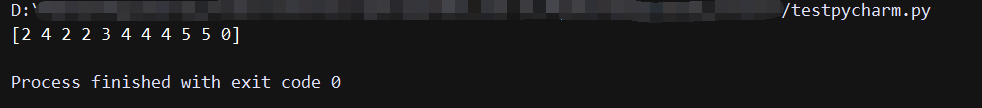可以看出， 打印的结果，和num的维度是一样的，1×11 接下来，再依次解释结果[2 4 2 2 3 4 4 4 5 5 0]中的值是怎么来的： 2：是num=[2,6,2,1,3,7,9,8,12,15,-1]中的第一个2，因为1.0<=2<2.5，这个[1.0，2.5）（默认right=False，左闭右开）是bins = [0.0,1.0,2.5,4.0,12]的第二个区间，所以第一个数为2； 4：同理，4.0<=4<12，在bins = [0.0,1.0,2.5,4.0,12]的第四个区间[4.0，12)，所以第二个数为4； …（依次同理） 再说一下，特殊一点的，比如， num=[2,6,2,1,3,7,9,8,12,15,-1]中的15：因为12<=15，位于(12,+∞）的第5区间，所以15对应的结果为5； num=[2,6,2,1,3,7,9,8,12,15,-1]中的-1：因为-1<2，位于(-∞,0.0）的第0区间，所以-1对应的结果为0。
最后，得出结论，np.digitize（x, bins, right=False）也就是将数组/矩阵x按照bins的区间，将x转为相同维度的整数，整数取值为[0,len(bins)]，具体取哪个整数值，取决于你定的bins区间和x对应位置的值的大小关系。
所以，在做图像处理的时候，np.digitize（x, bins, right=False）有一个很好的用途就是它可以对灰度级数进行一个降级：
import cv2
import numpy as np
bins = np.array([0, 16, 32, 48, 64, 80, 96, 112, 128, 144, 160, 176, 192, 208, 224, 240, 255])
inds = np.digitize(grayImage,bins)

输入的是256级的灰度图像，使用np.digitize通过bins区间进行区分，可将其降为0-16级的灰度，减少后续的图像计算，我也是在做图像处理这一块儿的时候碰到的，=。=。
2、np.flatten()
碰到这个函数的原因，主要是用Python绘图的时候，多维数组（不知道是不是这么说，反正是很多个括号，下面例子里有），会报错，不能绘图，所以网上查了下，找到了这个函数，能用，暂时先说如何使用，不深究其源码实现。
例子如下：
import cv2
import numpy as np
import matplotlib.pyplot as plt

a = [[],[],[],[],[],[],[]]
plt.plot(a,'k--')
plt.show()

if cv2.waitKey(0) & 0xFF ==ord('q'):
cv2.destroyAllWindows()

这样直接绘图，是要报错的，报错如下：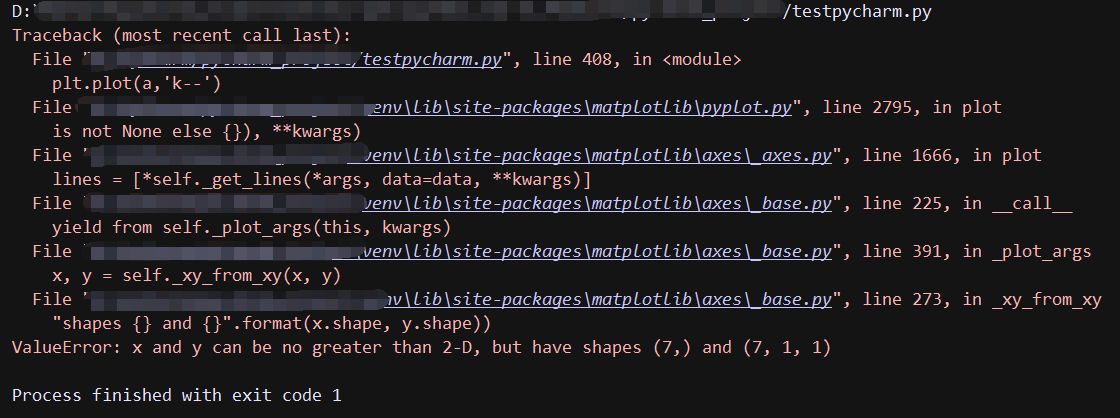报错原因就是方括号多了（不知道是不是叫多维数组）的原因，然后我就从网上找到去除方括号的办法，就是用python的内置函数np.flatten()，代码如下：
import cv2
import numpy as np
import matplotlib.pyplot as plt

a = [[],[],[],[],[],[],[]]
#np.flatten()不能直接作用于list，只能作用于array，所以需转换一下类型
a = np.array(a).flatten()
#再把类型转换回来
a = list(a)
print(a)
print(type(a))

plt.plot(a,'k--')
plt.show()

if cv2.waitKey(0) & 0xFF ==ord('q'):
cv2.destroyAllWindows()

代码执行结果：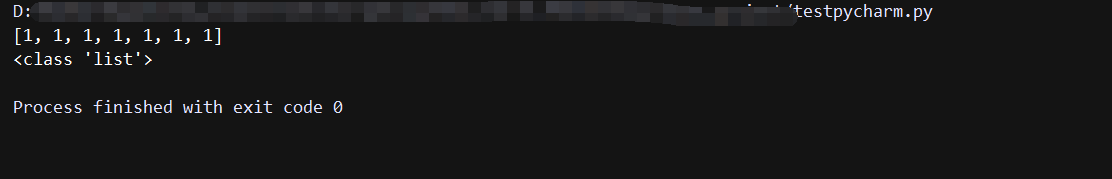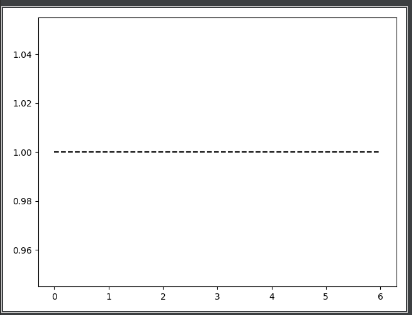去除方括号后，运行就没问题。
需要注意的是，np.flatten()不能直接作用于list，只能作用于array，所以再使用前需要进行类型转换，代码注释中也有说明。
另外，从网上也查到，np.ravel()也有类似功能，区别在于使用np.ravel()返回的是视图，会对原数据修改；使用np.flatten()返回的是拷贝，不会对原数据修改。
两者区别详见：https://blog.csdn.net/hanshuobest/article/details/78882425，就不另外验证了。
3、Python数据的强制类型转换：data = data.astype(np.uint8)
查看数据类型：
print(type(test_result))
print(test_result.dtype)

type(var)表示var是list、tuple还是np.array的那种数据类型； var.dtype表示var是int、float的那种数据类型。
强制类型转换
1、data = data.astype(np.uint8)的格式，括号内为你想强制转换为的数据类型。
lbp = local_binary_pattern(grayImage, n_points, radius, method='default')
# 类型转换，lbp为int32，将其转为uint8，为了符合cv2.calcHist()的数据类型要求
lbpInt = lbp.astype(np.uint8)

2、data = int(data) data = float(data) data = np.float32(data) 第二种方法没试过，但估计可以。
4、保留矩阵数据的小数点位数设置：mat= np.around(mat,4)
opencv_hist = originLBP(grayImage,radius = 1,n_points = 8)
opencv_hist = opencv_hist.flatten()#np.array(opencv_hist).flatten()
print(opencv_hist)

原始数据打印如下（部分）：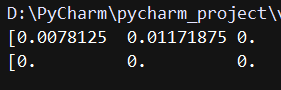加入opencv_hist = np.around(opencv_hist,4)后：
opencv_hist = originLBP(grayImage,radius = 1,n_points = 8)
opencv_hist = opencv_hist.flatten()#np.array(opencv_hist).flatten()
#只保留小数点后四位
# opencv_hist = np.around(opencv_hist,4)
print(opencv_hist)

打印数据如下（部分）：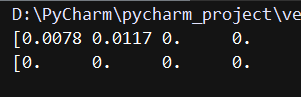mat= np.around(mat,4)，括号内的数字为矩阵元素打算保留的小数点位数。 mat= np.around(mat,num)和一般数据保留小数点位数的方法一致：data=round(data,num)。
展开全文Python numpy
• 这个 好像没什么好些的，但是还是记录下来吧 直接来代码  t = np.linspace(0, 2*np.pi, 100) ...y = (13 * np.cos(t) - 5 * np.cos(2*t) - 2 * np.cos(3*t) - np.cos(4*t))/1.5 plt.plot(x, y, 'r-', linewidt...
这个 好像没什么好些的，但是还是记录下来吧   直接来代码
t = np.linspace(0, 2*np.pi, 100)
x = (16 * np.sin(t) ** 3)/1.5
y = (13 * np.cos(t) - 5 * np.cos(2*t) - 2 * np.cos(3*t) - np.cos(4*t))/1.5
plt.plot(x, y, 'r-', linewidth=2)
#plt.fill(x, y,'red')
m = (16 * np.sin(t) ** 3)/2-13
n = (13 * np.cos(t) - 7 * np.cos(2*t) - 1* np.cos(3*t) - np.cos(4*t))/2
plt.plot(m, n, 'red', linewidth=2)
plt.fill(m, n,'red')#'#DC143C' '#FF1493'  'darkred''#8B0000','firebrick':'#B22222',
a=np.arange(-30,20)
b=0.5*a+4
plt.plot(a,b,'red', linewidth=3)
plt.fill(x, y,'red')
c=np.arange(19,21)
d=-2*c+52.8
plt.plot(c,d,'red', linewidth=3)
plt.fill(x, y,'red')

p=np.arange(-30,-26)
q=p*0-11
plt.plot(p,q,'red', linewidth=3)

r=np.arange(-11,-8)
s=r*0-30
plt.plot(s,r,'red', linewidth=3)
plt.grid(True)
plt.show()

这段代码就是画心形线，当然略有不同。
t = np.linspace(0, 2*np.pi, 100) x = (16 * np.sin(t) ** 3)/1.5 y = (13 * np.cos(t) - 5 * np.cos(2*t) - 2 * np.cos(3*t) - np.cos(4*t))/1.5
这样的t = np.linspace(0, 2*np.pi, 100)可以换成t = np.linspace(0, 2*math.pi, 100)  但是下面的就不可以换成x = (16 * math.sin(t) ** 3)/1.5 。
好吧 仔细一想当然不同了 。用numpy的时候 还是用 np里的函数吧 。

展开全文python
• python内置函数 Python内置(built-in)函数随着python解释器的运行而创建。在Python的程序中，你可以随时调用这些函数，不需要定义。 Built-in Functions abs() dict() help() m...python
• python内置的enumerate函数将一个可遍历的数据对象(如列表、元组或字符串)组合为一个索引序列，同时列出数据和数据下标，一般用在 for 循环当中。 sequence: 序列、迭代器或者其他支持迭代的对象 start: 下标起始...
• 1.python内置函数目录 2.python内置函数使用介绍 (1)map (2)arange (3)range (4)linspace等差数列 (5)filter过滤序列 (6)ascii输出ASCII码 (7)ord输出Unicode值 1.python内置函数目录 abs() #返回绝对值,...python
• pytorch与tensorflow API速查表 方法名称 pytroch ... np.clip(x, min, max) 取最大值 torch.max(x, dim) tf.max(x, axis) np.max(x, axis) 取最小值 torch.min(x, dim)
• TensorFlow - tf.reshape 函数 & Pytorch - torch.reshape 函数 TensorFlow tf.reshape( tensor, shape:[int, …], name=None ) Pytorch torch.reshape(self: Tensor, shape: Tuple[int, …]) ...
• 众所周知，Python有许多内置函数（例如min(),max(),sum()），Numpy也有自己的内置函数np.min(),np.max(),np.sum()）。由于Numpy的函数是在编译码中执行操作的，所以Numpy的操作计算得更快一些 接下来比较Numpy内置...python numpy
• 内置函数 文章目录内置函数array(创建一个数组)lenth(获取长度)linspace(创建直线)zeros(创建一个全为0的矩阵)ones(创建一个全为1的矩阵) array(创建一个数组) 格式：np.arange([start],stop,[step],dtype=None) ...numpy python 机器学习
• ## numpy 内置函数总结

千次阅读 多人点赞 2016-07-22 11:06:50
np的数组创建函数（ndarray） 函数 说明 np.array 将输入数据（列表，元组，数组或其他序列类型）转换为ndarray。要么推断出dtype，要么显示指定dtype。默认直接复制输入数据。比如：arr1 = np.array([1....numpy
• 目录 一、zeros 二、ones 三、full ...使用ndarray提供的内置函数可以快速，简单的创建需要的ndarray数组。 一、zeros import numpy as np #np.zeros 创建用0填充的ndarray arr = np.zero...
• 其中用到了内置函数 concat，它是以 Series 对象组成的列表为输出，当参数 axis 的值为 1 的时候把他们一行一行从左到右的组合成一个 DataFrame 的对象，。然后使用方法 cov 得到这三个行之间的一个相关矩阵。 ...
• 参考链接: 内置函数:any(iterable) 参考链接: numpy.ndarray.any 参考链接: numpy.any
• 最近看到python的内置函数bin(),想到也许一些问题转换成二进制后解决起来说不定更加容易一些。 bin()函数说明：http://www.runoob.com/python/python-func-bin.html 举个简单的栗子吧: 如果盒子中有红黄蓝(R,Y,B)...python 二进制 bin
• 本笔记整理自 udacity 课程，版权归 udacity ...import numpy as np 在此引入一次，下面直接使用 np 使用 np.zeros(shape) 创建 X = np.zeros((3,4)) print() print('X = \n', X) print() print('X has dimen...Python ndarray
• 内置函数的数据聚合 实验环境 Python 3.6.1 PyCharm 实验内容 数据聚合函数的应用 代码部分 import numpy as np import pandas as pd 1.创建六行五列的随机整数DataFrame对象，列索引为：A-E，行索引为：1,2，3,4,...
• 按照函数进行分组 实验环境 Python 3.6.1 Jupyter 实验内容 数据聚合函数的应用 代码部分 import numpy as np import pandas as pd 1.创建三行四列的随机整数DataFrame对象，整数范围1-6 df1 = pd.DataFrame(np....
• import numpy as np a = np.array([1, 2, 3]) print("a: {}".format(a)) b = a.tolist() print("b: {}".format(b)) c = sum(b) print("sum: {}".format(c)) d = sum(b, 2) print("sum extra add 2: {}".format(d))shell...